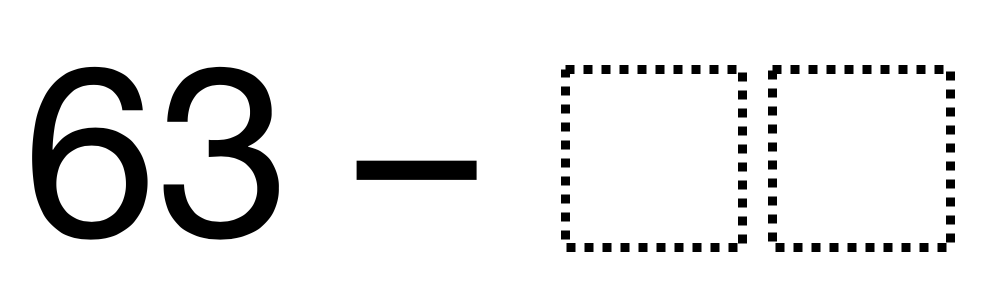# Subtraction without Regrouping

Directions: Using the digits 1 to 9, at most one time each, fill in the boxes so that you would not need to regroup when you subtract. Make sure your number is less than 63.

Extension: Explain why you do NOT need to regroup using your number.### Hint

What is a number you can take away from 3?

The student puts in a number less than 63 and contains a 0, 1, 2, or 3 in the ones place.

Source: Chase Orton

## Time Twister

Directions: Using the digits 0 to 9, at most one time each, create three different …

1.Does not come up as a PDF

2.3.4.5.63 – 51

6.7.Michelle Ammirati

63-31

8.63-21

9.63 – 32

10.Shalonda Woods-Hornsby

63-53

•11.63-42

12.63-12

13.14.I wonder if anyone from a middle school background wrote 63 – (-5). It meets the qualifications.

15.63-12, since there are less ones in 12 then 63 i do not have to regroup

16.63-41 would not require regrouping because the number to subtract has a value in both the ones and tens place that is less than the greater number. 1 < 3 and 4 < 6 This way no regrouping is required to solve the problem.

17.63-23. No need to regroup since there are two 3’s in the ones place that will give a 0 and in the tens place you have 6-2, which will give a 4

18.Paul Nelson Bennett

I need to make sure i am subtracting numbers less than the number in each place value.

19.63-23
Ones place must have a number that is the same or less value so you won’t have to rename/regroup

20.63-52

21.63-21

22.23.63-1=62

24.Shannon Wirtzfeld

63-52=11

25.63-52=11

The number must be smaller than 63 and have a smaller number than 3 in the ones place in order to have no regrouping.

26.63-03 will not need regrouping because subtracting 3 form 3 in the ones place value gives a value of zero and leave 6 in the tens place

27.63-52=11
The numbers are smaller than the numbers in the tens and ones column.

28.63-10=53
No regrouping is required because the number on top is bigger or equal than the number that is being taken away.